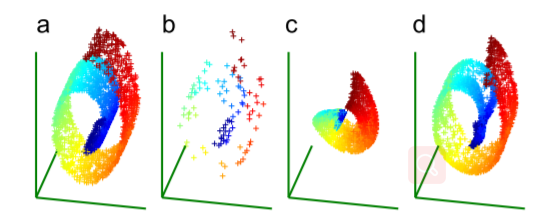# Multi-output regression on the output manifold### Abstract

Multi-output regression aims at learning a mapping from an input feature space to a multivariate output space. Previous algorithms define the loss functions using a fixed global coordinate of the output space, which is equivalent to assuming that the output space is a whole Euclidean space with a dimension equal to the number of the outputs. So the underlying structure of the output space is completely ignored. In this paper, we consider the output space as a Riemannian submanifold to incorporate its geometric structure into the regression process. To this end, we propose a novel mechanism, called locally linear transformation (LLT), to define the loss functions on the output manifold. In this way, currently existing regression algorithms can be improved. In particular, we propose an algorithm under the support vector regression framework. Our experimental results on synthetic and real-life data are satisfactory.

Type
Publication
Pattern Recognition